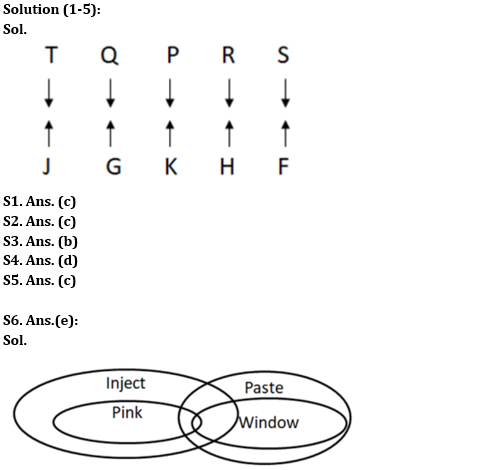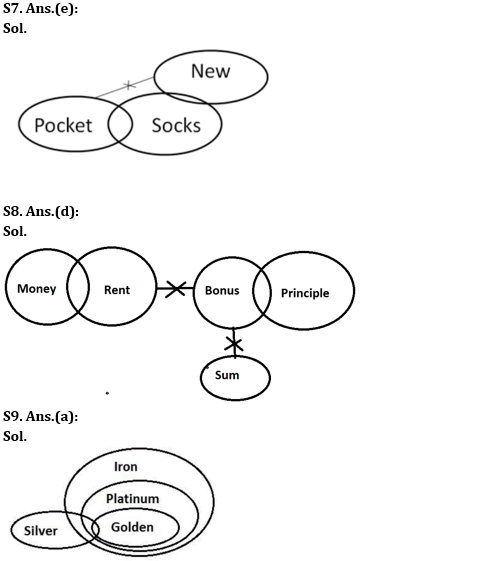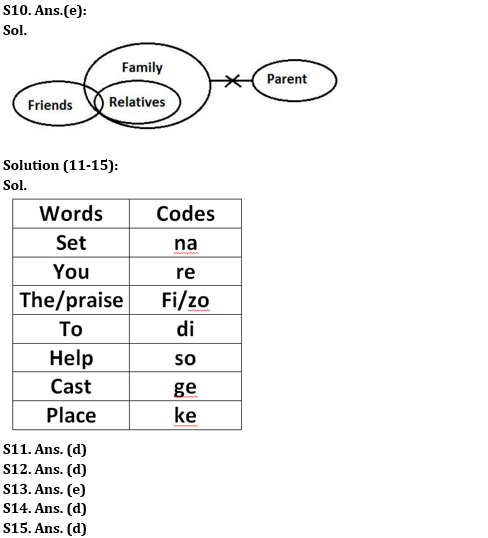Latest Banking jobs   »   Reasoning Ability Quiz For RBI Grade...

# Reasoning Ability Quiz For RBI Grade B/ ECGC PO/ SIDBI Grade A Prelims 2022- 3rd May

Directions (1-5): Study the following information carefully and answer the questions accordingly.
Ten people are sitting in two parallel rows. In Row 1 – P, Q, R, S, and T are sitting facing the south direction and in Row 2 – F, G, H, J, and K are sitting facing the north direction but not necessarily in the same order. The people sit in row 1 are facing the people sit in row 2 and vice versa. T sits third to the right of R. The one who sits opposite T, sits second to the left of K. F sits to the right of K. Two people sit between F and G who does not sit opposite T. P sits second to the right of S. J does not sit opposite R.

Q1. How many people sit between J and K?
(a) Three
(b) Two
(c) One
(d) Either One or Two
(e) None

Q2. Who among the following sits opposite S?
(a) J
(b) K
(c) F
(d) H
(e) None of these

Q3. Who among the following sits to the right of Q?
(a) R
(b) T
(c) S
(d) P
(e) None of these

Q4. Find the correct statement/statements from the following.
1. H sits to the right of F.
2. P sits opposite G.
3. J sits diagonally opposite S.
(a) Only 2
(b) Both 1 and 3
(c) Both 2 and 3
(d) Only 3
(e) None is incorrect

Q5. Four from the following five are similar in a certain way and thus form a group. Find out the one who does not belong to that group.
(a) T
(b) J
(c) R
(d) F
(e) S

Directions (6-10): In each of the questions below, three statements are given followed by two conclusions numbered I and II. You have to take the given statements to be true even if they seem to be at variance with commonly known facts. Read all the conclusions and then decide which of the given conclusions logically follows from the given statements disregarding commonly known facts.

Q6. Statements: All Windowsare Pastes. All Pinks are Injects. Only a few Pink are Windows.
Conclusions:
I. All Paste being Inject is a possibility.
II. Some Pastes are Pink.

(a) If only conclusion I follows.
(b) If only conclusion II follows.
(c) If either conclusion I or II follows.
(d) If neither conclusion I nor II follows.
(e) If both conclusions I and II follow.

Q7. Statements: Some Pockets are Socks. Only a few Socks are New. No New is a Pocket.
Conclusions:
I. All New being Socks is a possibility.
II. Some Socks are not Pockets.

(a) If only conclusion I follows.
(b) If only conclusion II follows.
(c) If either conclusion I or II follows.
(d) If neither conclusion I nor II follows.
(e) If both conclusions I and II follow.

Q8. Statements: Some money is rent. Some bonus is principal. No sum is bonus. No rent is bonus.
Conclusions:
I. No money is Principle.
II. All rent being sum is not possible.

(a) If only conclusion I follows.
(b) If only conclusion II follows.
(c) If either conclusion I or II follows.
(d) If neither conclusion I nor II follows.
(e) If both conclusions I and II follow.

Q9. Statements: Only a few Silvers are Golden. All Golden are Platinum. All Platinum are Iron.
Conclusions:
I. Some Platinums are Silver.
II. Some Iron are not Silver.

(a) If only conclusion I follows.
(b) If only conclusion II follows.
(c) If either conclusion I or II follows.
(d) If neither conclusion I nor II follows.
(e) If both conclusions I and II follow.

Q10. Statements: Only a few Friends are Relatives. All Relatives are Family. No Family is Parent.
Conclusions:
I. Some Friends are not Relatives.
II. No Relatives are Parents.

(a) If only conclusion I follows.
(b) If only conclusion II follows.
(c) If either conclusion I or II follows.
(d) If neither conclusion I nor II follows.
(e) If both conclusions I and II follow.

Directions (11-15): Study the following data carefully and answer the questions accordingly.
In an artificial language,
‘Set you the praise’ is written as ‘re fi zo na’,
‘Help to set you’ is written as ‘so re na di’,
‘Place cast to set the praise’ is written as ‘ge fi na di ke zo’,
‘Help to cast’ is written as ‘di so ge’.

Q11. Which of the following words are coded as ‘re di so’?
(a) Praise you to
(b) To cast you
(c) Set you to
(e) Can’t be determined

Q12. What will be the code for ‘place set you’?
(a) so di na
(b) ke na di
(c) na di so
(d) ke re na
(e) Cannot be determined

Q13. What will be the code for ‘praise’?
(a) ge
(b) re
(c) zo
(d) fi
(e) Cannot be determined

Q14. Which of the following words is coded as ‘so’?
(a) Cast
(b) To
(c) Set
(d) Help
(e) None is correct

Q15. What will be to code for ‘place’?
(a) di
(b) zo
(c) na
(d) ke
(e) Cannot be determined

Solutions